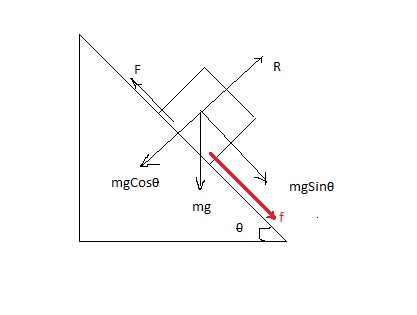# A crate of mass 11 \ kg is pulled up a rough incline whit an initial speed of 1.48 \ m/s. The...

## Question:

A crate of mass {eq}11 \ kg {/eq} is pulled up a rough incline whit an initial speed of {eq}1.48 \ m/s {/eq}. The pilling force is {eq}108 \ N {/eq} parallel to the incline, which makes an angle of {eq}19^o {/eq} with the horizontal. The coefficient of kinetic friction is {eq}0.400 {/eq}, and the crate is pulled {eq}5.06 \ m. {/eq}

a. How much work is done by the gravitational force on the crate?

b. Determine the increase in internal energy of the crate-incline system owing to friction.

c. How much work is done by the {eq}108 \ N {/eq} force on the crate?

d. What is the change in kinetic energy of the crate?

e. What is the speed of the crate after being pulled {eq}5.06 \ m {/eq}?

## Work-done and Newton's Law:

The work-done due to the constant force is defined as the energy that is provided to the object during the displacement. The mathematical expression of the work-done is as follows:

{eq}\rm W = \vec{F} \cdot \vec{S} \\ W = FS\cos\theta {/eq}

• F is the force
• S is the displacement
• {eq}\rm \theta {/eq} is the angle between the force and the displacement.Given data:

• Mass of the crate {eq}\rm (m) = 11 \ kg {/eq}
• Initial speed of the crate {eq}\rm (u) = 1.48 \ m/s {/eq}
• Force applied on the crate {eq}\rm (F) = 108 \ N {/eq}
• Angle of the plane {eq}\rm (\theta) = 19^\circ {/eq}
• Displacement along the plane {eq}\rm (S) = 5.06 \ m {/eq}

(a)

The gravitational force acts on the crate is {eq}\rm F_{g} = mg\sin\theta \\ F_{g} = 11 \times 9.8 \times \sin19^\circ \\ F_{g} = 35.09 \ N {/eq}

Since the displacement is along the plane or we can say in the direction of force, therefore the work done can be written as

{eq}\rm W_{g} = F_{g} \times S\cos0^\circ \\ W_{g} = F_{g} \times S\\ W_{g} = 35.09 \times 5.06 \\ W_{g} = 178 \ J {/eq}

(b)

The energy that is lost due to the friction will store in the form of internal energy of the object, therefore, change in the internal energy of the object will be equal to the frictional energy.

{eq}\rm W_{f} = f \times S \\ W_{f} = (\mu \times mg\cos\theta) \times S \\ W_{f} = (0.4 \times 11 \times 9.8 \cos19^\circ) \times 5.06 \\ W_{f} = 206 \ J {/eq}

(c)

Now, the work done by the pulling force

{eq}\rm W_{F} = F \times S \\ W_{F} = 108 \times 5.06 \\ W_{F} = 546 \ J {/eq}

(d)

Applying the energy conservation,

{eq}\begin{align} \text{Initial kinetic energy + Work done by applied force} &= \text{Final kinetic energy + Potetnial energy + frictional energy} \\ \rm \dfrac{1}{2}mu^{2} + W_{F} &= \rm K_{f} + mgh + W_{f} \\ \rm (0.5 \times 11 \times 1.48^{2}) + 546 &= \rm K_{f} + (11 \times 9.8 \times S\sin\theta) + 206 \\ \rm 12.05 + 546 &= \rm K_{f} + (11 \times 9.8 \times 5.06 \sin19^\circ) + 206 \\ \rm K_{f} &= \rm 174 \ J \\ \end{align} {/eq}

(e)

The final speed of the crate would be

{eq}\rm K_{f} = \dfrac{1}{2} mv^{2} \\ 174 = \dfrac{1}{2} (11)v^{2} \\ v = 5.62 \ m/s {/eq}